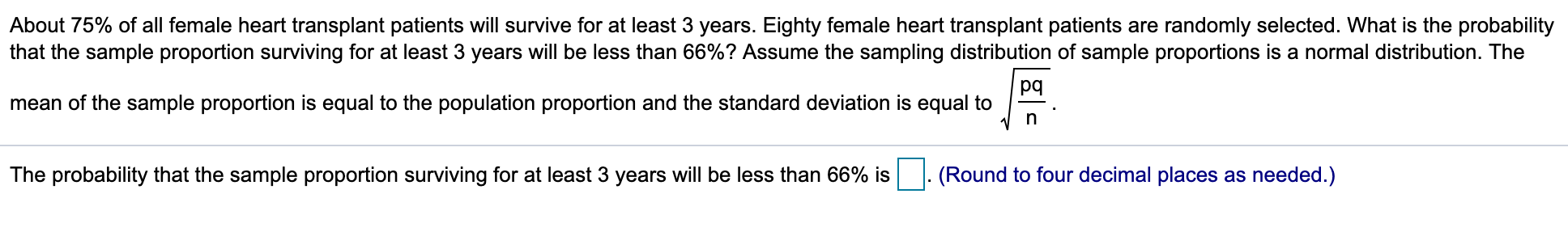# About 75% of all female heart transplant patients will survive for at least 3 years. Eighty female heart transplant patients are randomly selected. What is the probabilitythat the sample proportion surviving for at least 3 years will be less than 66%? Assume the sampling distribution of sample proportions is a normal distribution. Thepqmean of the sample proportion is equal to the population proportion and the standard deviation is equal toThe probability that the sample proportion surviving for at least 3 years will be less than 66% is(Round to four decimal places as needed.)

Question
6 views

I don't understand how to solve thishelp_outlineImage TranscriptioncloseAbout 75% of all female heart transplant patients will survive for at least 3 years. Eighty female heart transplant patients are randomly selected. What is the probability that the sample proportion surviving for at least 3 years will be less than 66%? Assume the sampling distribution of sample proportions is a normal distribution. The pq mean of the sample proportion is equal to the population proportion and the standard deviation is equal to The probability that the sample proportion surviving for at least 3 years will be less than 66% is (Round to four decimal places as needed.) fullscreen
check_circle

Step 1

It is given that the proportion of all female heart transplant patients will survive for...

### Want to see the full answer?

See Solution

#### Want to see this answer and more?

Solutions are written by subject experts who are available 24/7. Questions are typically answered within 1 hour.*

See Solution
*Response times may vary by subject and question.
Tagged in

### Other